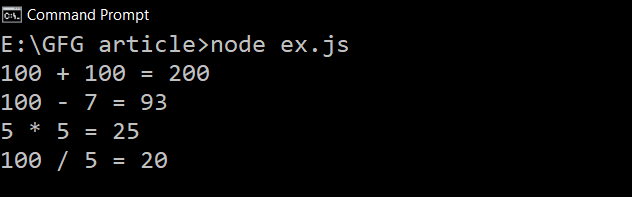Related Articles

# Difference between First-Class and Higher-Order Functions in JavaScript

• Last Updated : 01 Jul, 2021

First-Class Function: A programming language is said to have First-class functions if functions in that language are treated like other variables. So the functions can be assigned to any other variable or passed as an argument or can be returned by another function.
JavaScript treat function as a first-class-citizens. This means that functions are simply a value and are just another type of object.

Example: Let us take an example to understand more about the first-class function.

## Javascript

 `const Arithmetics = {``    ``add:(a, b) => {``        ``return` ``\${a} + \${b} = \${a+b}`;``    ``},``    ``subtract:(a, b) => {``        ``return` ``\${a} - \${b} = \${a-b}```    ``},``    ``multiply:(a, b) => {``        ``return` ``\${a} * \${b} = \${a*b}```    ``},``    ``division:(a, b) => {``        ``if``(b!=0) ``return` ``\${a} / \${b} = \${a/b}`;``        ``return` ``Cannot Divide by Zero!!!`;``    ``}` `}` `console.log(Arithmetics.add(100, 100));``console.log(Arithmetics.subtract(100, 7));``console.log(Arithmetics.multiply(5, 5));``console.log(Arithmetics.division(100, 5));`

Output: In the above program, functions are stored as a variable in an object.First Class Function

Higher-Order Function: A function that receives another function as an argument or that returns a new function or both is called Higher-order functions. Higher-order functions are only possible because of the First-class function.

Let’s take some examples to understand better:

Example 1: Functions returning another function.

## Javascript

 `const greet =  ``function``(name){``    ``return` `function``(m){``  ` `        ``console.log(`Hi!! \${name}, \${m}`);``    ``}``}` `const greet_message = greet(``'ABC'``);``greet_message(``"Welcome To GeeksForGeeks"``)`

Note: We can also call the function like this also — greet(‘ABC’)(‘Welcome To GeeksForGeeks’), It will also give the same output.

Output:

`Hi!! ABC, Welcome To GeeksForGeeks`

Example 2: Passing Function as an argument.

## Javascript

 `function` `greet(name){``    ``return` ``Hi!! \${name} `;``}` `function` `greet_name(greeting,message,name){``       ``console.log(`\${greeting(name)} \${message}`);``}` `greet_name(greet,``'Welcome To GeeksForGeeks'``,``'JavaScript'``);`

Note: The function that we pass as an argument to another function is called the callback function.

Output:

`Hi!! JavaScript  Welcome To GeeksForGeeks`

Note: Functions such as filter(),map(),reduce(),some() etc, these all are example of Higher-Order Functions.

Key Differences between First-Order Function and Higher-Order Function:-

Hey geek! The constant emerging technologies in the world of web development always keeps the excitement for this subject through the roof. But before you tackle the big projects, we suggest you start by learning the basics. Kickstart your web development journey by learning JS concepts with our JavaScript Course. Now at it’s lowest price ever!

My Personal Notes arrow_drop_up